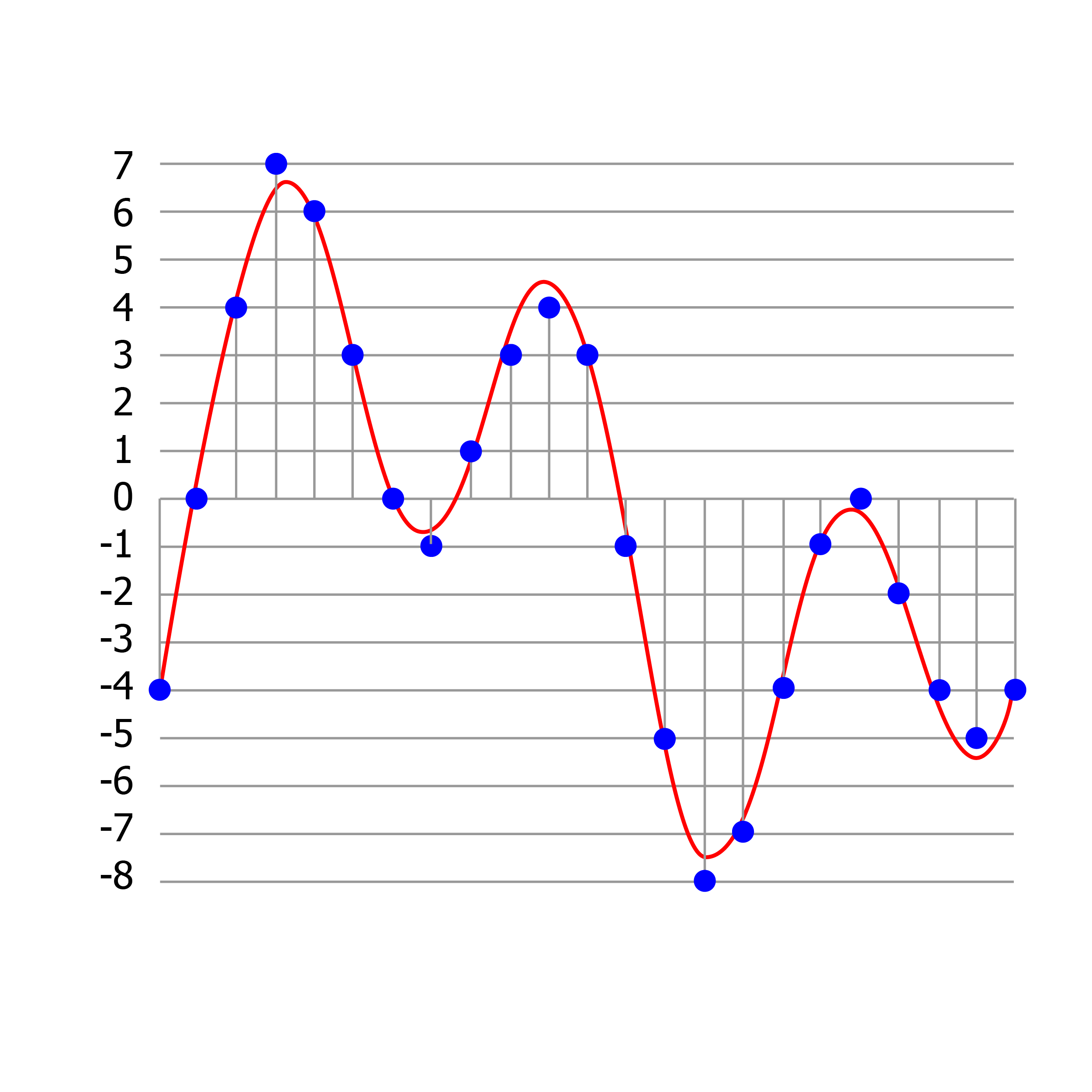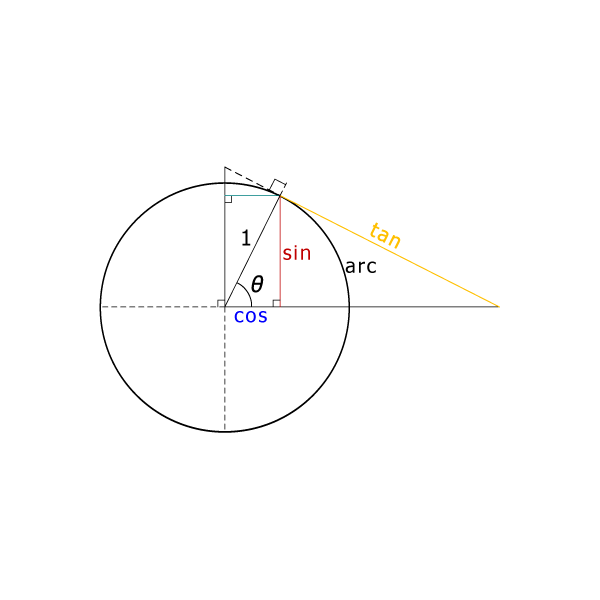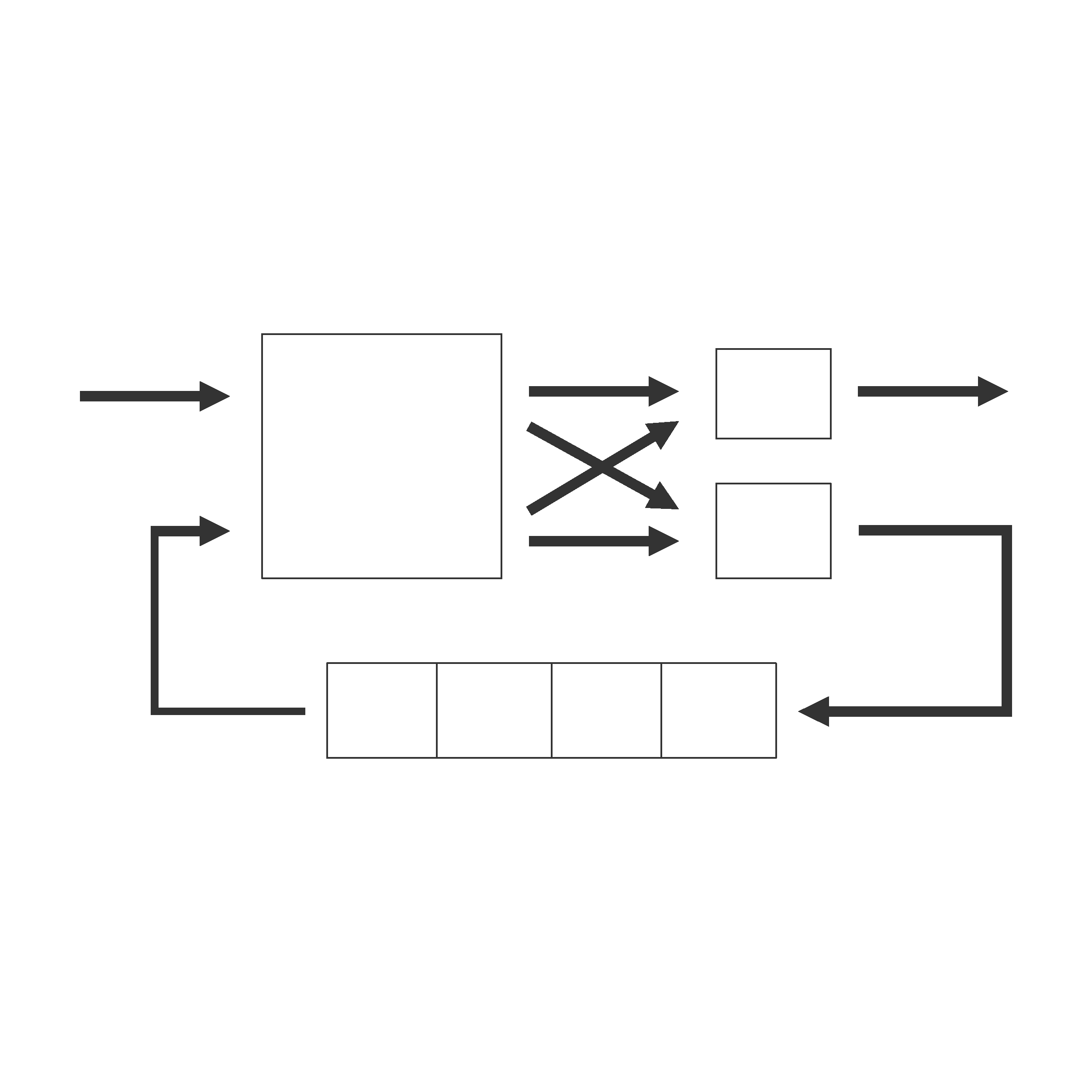#### Welcome to hlslibs!

HLSLibs is a free and open set of libraries implemented in standard C++ for bit-accurate hardware and software design. The goal of HLSLibs is to create an open community for exchange of knowledge and IP for HLS (High-Level Synthesis) that can be used to accelerate both research and design. The libraries are targeted to enable a faster path to hardware acceleration by providing easy-to-understand, high-quality fundamental building blocks that can be synthesized into both FPGA and ASIC. HLSLibs are delivered as an open-source project on GitHub under the Apache 2.0 license and contributions are welcome.#### AC Datatypes

The Algorithmic C datatypes include a numerical set of datatypes and an interface datatype for modeling channels in communicating processes in C++. The numerical datatypes provide an easy way to model static bit-precision with minimal runtime overhead. They include bit-accurate integer, fixed-point, floating-point and complex datatypes. The numerical datatypes were developed in order to provide a basis for writing bit-accurate algorithms to be synthesized into hardware.

The Algorithmic C (AC) datatypes include a numerical set of datatypes and an interface datatype for modeling channels in communicating processes in C++. The numerical datatypes provide an easy way to model static bit-precision with minimal runtime overhead. They include bit-accurate integer, fixed-point, floating-point and complex datatypes. The numerical datatypes were developed in order to provide a basis for writing bit-accurate algorithms to be synthesized into hardware. The Algorithmic C Datatypes are used in Catapult High-Level Synthesis, a tool that generates optimized RTL from algorithms written as sequential ANSI-standard C/C++ specifications.

Operators and methods on both the integer and fixed-point types are clearly and consistently defined so that they have well defined simulation and synthesis semantics. They enable algorithm, system and hardware designers to precisely model bit-true behavior in C++ specifications while accelerating simulation speeds by 10-200x faster versus alternate bit-accurate integer and fixed-point datatypes. The bit-accurate complex and floating-point types are unique in that they are not available in alternative datatype packages such as SystemC. Bit-accurate complex types are important because they allow computation of additive and multiplicative operations on a mixture of complex operands based on bit-accurate integer and fixed-point types and return the require type so that results are represented without any loss of precision.

#### The advantages of the AC datatypes over the existing integer and fixed-point datatypes are the following:

Arbitrary-Length: This allows a clean definition of the semantics for all operators that is not tied to an implementation limit. It is also important for writing general IP algorithms that don’t have artificial (and often hard to quantify and document) limits for precision. For example, Catapult provides generic math functions such as division and square root for arbitrary-length datatypes.

Precise and Consistent Definition of Semantics: Special attention has been paid to define and verify the simulation semantics and to make sure that the semantics are appropriate for synthesis. No simulation behavior has been left to compiler dependent behavior. Also, asserts have been introduced to catch invalid code during simulation. There is consistency of semantics across datatypes. Whenever possible, the return type of operators does not loose precision.

Correctness: The simulation and synthesis semantics have been verified for many size combinations using a combination of simulation and equivalence checking.

Simulation Speed: The implementation of ac_int uses sophisticated template specialization techniques so that a regular C++ compiler can generate optimized assembly language that will run much faster than the equivalent SystemC datatypes. For example, ac_int of bit widths in the range 1 to 32 can run 100x faster than the corresponding sc_bigint/sc_biguint datatype and 3x faster than the corresponding sc_int/sc_uint datatype.

Compilation Speed and Smaller Executable: Code written using ac_int datatypes compiles 5x faster even with the compiler optimizations turned on (required to get fast simulation). It also produces smaller binary executables.

#### WHAT YOU GET

###### The zipped folder contains a reference manual, release notes, header files and a python script for pretty printing of the AC Datatypes in the gdb debugger.

• ac_channel.h - Header to model streaming communication between blocks in C++.
• ac_complex.h - Header for complex (real, imaginary) datatype.
• ac_fixed.h - Header for bit-accurate fixed-point types.
• ac_float.h - Header for bit-accurate floating-point types. The mantissa is an ac_fixed (two's complement) and the exponent is an ac_int.
• ac_int.h - Header for bit-accurate integer types. It also provides other utility functions and contains the base classes to implement arbitrary length arithmetic.

• ac_sc.h - Header containing conversion function to and from SystemC datatypes and tracing function to use the AC Datatypes within SystemC.
• ac_pp.py - Python script for pretty printing of the AC Datatypes in the gdb debugger
• Reference Manual - Overview of Algorithmic C Datatypes
• Release Notes - Algorithmic C (AC) Datatypes Release Notes Current Software Version#### AC Math

The Algorithmic C Math Library contains synthesizable C++ functions commonly used in Digital Signal Processing applications. The functions use the Algorithmic C data types and are meant to serve as examples on how to write parameterized models and to facilitate migrating an algorithm from using floating-point to fixed-point arithmetic where the math functions either need to be computed dynamically or via lookup tables or piecewise linear approximations. The library includes basic math functions (reciprocal, log, exponent, square-root, sin/cos/tan, etc) as well as a matrix storage class and linear algebra functions like multiplication, determinant, Cholesky Inverse/Decomposition, etc. Each function comes with a unit test to demonstrate usage and measurement of errors due to approximations.

The Algorithmic C Math Library (ac_math) contains synthesizable C++ functions commonly used in Digital Signal Processing applications. The functions use the Algorithmic C data types and are meant to serve as examples on how to write parameterized models and to facilitate migrating an algorithm from using floating-point to fixed-point arithmetic where the math functions either need to be computed dynamically or via lookup tables or piecewise linear approximations. The input and output arguments of the math functions are parameterized so that arithmetic may be performed at the desired fixed point precision and provide a high degree of flexibility on the area/performance trade-off of hardware implementations obtained during Catapult synthesis. The hardware implementations produced by Catapult on the math functions are bit accurate. Simulation of the RTL can thus be easily compared to the C++ simulation of the algorithm. This library also contains a 2-D matrix class (ac_matrix) which implements the most common matrix operations along with other Linear Algebra functions like Cholesky Decomposition.

#### Summary of Basic Math Functions:

Function Type  Function Call  Approximation Method Supported Data Types
ac_fixed ac_float ac_complex
Absolute Value  ac_abs()  N/A  Yes  Yes  No
Division  ac_div()  N/A  Yes  Yes  Yes
Normalization  ac_normalize()  N/A  Yes  No  Yes
Reciprocal  ac_reciprocal_pwl()  PWL  Yes  Yes  Yes
Logarithm Base e  ac_log_pwl()  PWL  Yes  No  No
ac_log_cordic()  CORDIC  Yes  No  No
Logarithm Base 2  ac_log2_pwl()  PWL  Yes  No  No
ac_log2_cordic()  CORDIC  Yes  No  No
Exponent Base e  ac_exp_pwl()  PWL  Yes  No  No
ac_exp_cordic()  CORDIC  Yes  No  No
Exponent Base 2  ac_pow2_pwl()  PWL  Yes  No  No
ac_exp2_cordic()  CORDIC  Yes  No  No
Square Root  ac_sqrt_pwl()  PWL  Yes  Yes  Yes
ac_sqrt()  N/A  Yes  No  No
Inverse Square Root  ac_inverse_sqrt_pwl()  PWL  Yes  Yes  Yes
Sine/Cosine  ac_sincos()  LUT  Yes  No  N/A
ac_cos_cordic()  CORDIC  Yes  No  N/A
ac_sin_cordic()  CORDIC  Yes  No  N/A
ac_sincos_cordic()  CORDIC  Yes  No  N/A
Tangent  ac_tan_pwl()  PWL  Yes  No  N/A
Inverse Trig  ac_atan_pwl()  PWL  Yes  No  N/A
ac_arccos_cordic()  CORDIC  Yes  No  N/A
ac_arcsin_cordic()  CORDIC  Yes  No  N/A
ac_arctan_cordic()  CORDIC  Yes  No  N/A
Shift Left/Right  ac_shift_left  N/A  Yes  No  Yes
ac_shift_right  N/A  Yes  No  Yes

#### AC Matrix Operators:

• Assignment: operator=()
• Comparison: operator!=(), operator==()
• Piecewise Subtraction: operator-(), operator-=()
• Piecewise Multiplication (nested loops): pwisemult()
• Matrix Multiplication: operator*()
• Matrix Transpose: transpose()
• Sum All Elements: sum()
• Scale All Elements: scale(value)
• Formatted Stream Output: ostream &operator<<()

#### Linear Algebra Functions:

• Matrix Multiplication
• Matrix Determinant
• Cholesky Decomposition
• Cholesky Inverse
• QR Decomposition#### AC DSP

The Algorithmic C Digital Signal Processing Library contains synthesizable C++ objects for common DSP operations like filters and Fast Fourier Transforms. The functions use a C++ class-based object design so that it is easy to instantiate multiple variations of objects into a more complex subsystem and utilizes the AC Datatypes for true bit-accurate behavior. The filter block set includes CIC Interpolation and Decimation, FIR filters, IIR filters, Moving Average Filters and Polyphase Interpolation/Decimation. The FFT block set includes various FFT architectures including Decimation-in-Time and Decimation-in-Frequency, various radix modes and various buffer architectures (memory-exchange, single-delay-feedback, in-place).#### MatchLib Connections

MatchLib Connections is a SystemC library implementing latency-insensitive channels for use by HLS tools. The Connections classes are parameterized and can be used to model hardware systems at a level of abstraction higher than RTL, while enabling HLS tools to generate efficient hardware. Connections also enable fast and accurate simulation and verification of hardware systems in SystemC prior to synthesis.

Additional documentation on the Connections library can be found here. Connections are a component of the MatchLib hardware component library, available here. For more documentation about compiling and running Connections components, see the MatchLib Documentation.